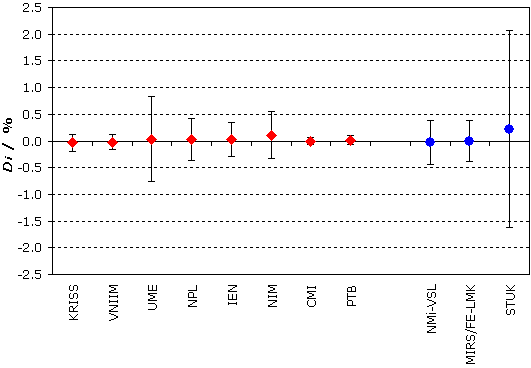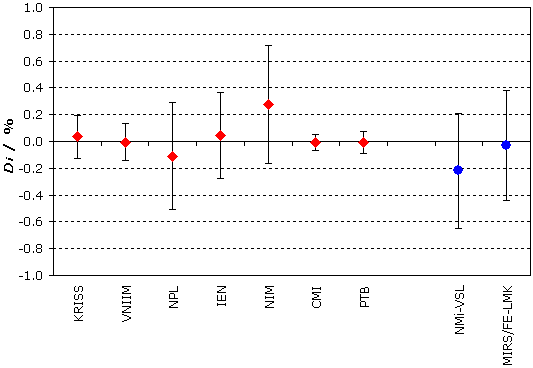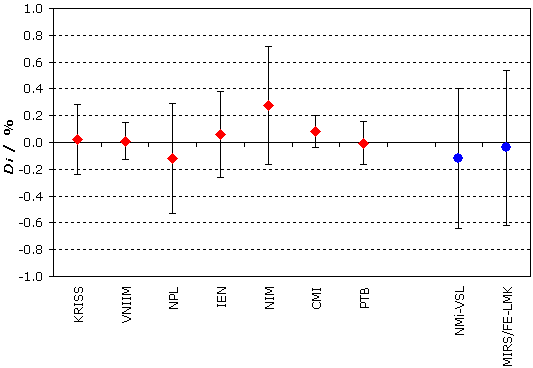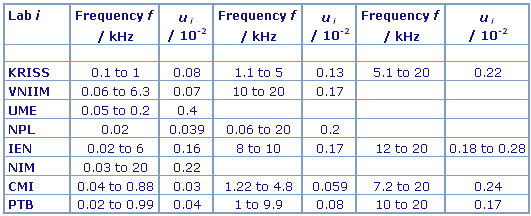Comparison
Comparison close
Results

CCEM.M-K1 and EUROMET.EM.M-K1

MEASURAND : Coil constant of a DC transfer standard

To view the Graph of equivalence showing degrees of equivalence Di and expanded uncertainty Ui (k = 2), click here.

CCEM.M-K1 and EUROMET.EM.M-K1

MEASURAND : Frequency dependence of the coil constant relative to DC
FREQUENCY : 50 Hz

Degrees of equivalence Di and expanded uncertainty Ui (k = 2)Red diamonds: participants in CCEM.M-K1
Blue circles: participants in EUROMET.EM.M-K1 only

CCEM.M-K1 and EUROMET.EM.M-K1

MEASURAND : Frequency dependence of the coil constant relative to DC
FREQUENCY : 1 kHz

Degrees of equivalence Di and expanded uncertainty Ui (k = 2)Red diamonds: participants in CCEM.M-K1
Blue circles: participants in EUROMET.EM.M-K1 only

CCEM.M-K1 and EUROMET.EM.M-K1

MEASURAND : Frequency dependence of the coil constant relative to DC
FREQUENCY : 5 kHz

Degrees of equivalence Di and expanded uncertainty Ui (k = 2)Red diamonds: participants in CCEM.M-K1
Blue circles: participants in EUROMET.EM.M-K1 only

Comparison
Comparison close
Results

CCEM.M-K1 and EUROMET.EM.M-K1

MEASURAND : Coil constant of a DC transfer standard

 Lab i Di Ui / 10-6 / 10-6 KRISS -7.8 8 VNIIM 0.8 2.2 NMIA 365 860 UME 15000 556 NPL 30.3 146 IEN -281 444 NIM -10.2 17.2 CMI 38.2 70 CENAM 1162 124 PTB 2.4 5.4 CENAMPTB 35.6 64 MIRS/FE-LMK -16.2 131 BNM-LCIE 3600 5000 NMi-VSL -1630 3000

In blue: participants in EUROMET.EM.M-K1.

CENAMPTB: measurements made at PTB with the CENAM flowing water instrument

Results are presented under A4 printable format in Summary Results (.PDF file).

CCEM.M-K1 and EUROMET.EM.M-K1

MEASURAND : Frequency dependence of the coil constant relative to DC
FREQUENCY : 50 Hz

 Lab i Di Ui / 10-2 / 10-2 KRISS -0.032 0.16 VNIIM -0.022 0.14 UME 0.036 0.8 NPL 0.028 0.4 IEN 0.028 0.32 NIM 0.108 0.44 CMI 0 0.06 PTB 0.016 0.08 NMi-VSL -0.03 0.41 MIRS/FE-LMK 0 0.39 STUK 0.22 1.84

In blue: participants in EUROMET.EM.M-K1.

Results are presented under A4 printable format in Summary Results (.PDF file).

CCEM.M-K1 and EUROMET.EM.M-K1

MEASURAND : Frequency dependence of the coil constant relative to DC
FREQUENCY : 1 kHz

 Lab i Di Ui / 10-2 / 10-2 KRISS 0.036 0.16 VNIIM -0.004 0.14 NPL -0.11 0.4 IEN 0.046 0.32 NIM 0.276 0.44 CMI -0.006 0.06 PTB -0.006 0.08 NMi-VSL -0.22 0.43 MIRS/FE-LMK -0.03 0.41

In blue: participants in EUROMET.EM.M-K1.

Results are presented under A4 printable format in Summary Results (.PDF file).

CCEM.M-K1 and EUROMET.EM.M-K1

MEASURAND : Frequency dependence of the coil constant relative to DC
FREQUENCY : 5 kHz

 Lab i Di Ui / 10-2 / 10-2 KRISS 0.02 0.26 VNIIM 0.01 0.14 NPL -0.12 0.41 IEN 0.06 0.32 NIM 0.276 0.44 CMI 0.08 0.12 PTB -0.005 0.16 NMi-VSL -0.12 0.52 MIRS/FE-LMK -0.04 0.58

In blue: participants in EUROMET.EM.M-K1.

Results are presented under A4 printable format in Summary Results (.PDF file).

Comparison
Comparison close
RMO comparison
 Metrology area, Sub-field Electricity and Magnetism, Magnetism Description Comparison of low frequency magnetic flux density Time of measurements 1998 - 2000 Status Approved for equivalence
 Final Reports of the comparisons Measurand Magnetic flux density Parameters Frequency: DC to 20 kHz Transfer device Two coil systems
 Comparison type Key Comparison Consultative Committee CCEM (Consultative Committee for Electricity and Magnetism) Conducted by EURAMET (European Association of National Metrology Institutes) RMO Internal Identifier EUROMET Project No 446 Comments Publication of CCEM.M-K1 and EUROMET.EM.M-K1 results on 27 May 2005 Coil constant of a DC transfer standard and its variation with frequency EUROMET.EM.M-K1 results are linked to those of CCEM.M-K1 for DC measurements, and at frequencies 50 Hz, 1 kHz, and 5 kHz Pilot institute PTB Physikalisch-Technische Bundesanstalt Germany Contact person K. Weyand +49 (0) 531 592 2240
First Name Last Name
wwww@ww.www +356719836 Institute 1 Institute 1 Khmelnitskiy
Pilot laboratory
PTB

Physikalisch-Technische Bundesanstalt, Germany, EURAMET

 CMI Czech Metrology Institute, Czechia, EURAMET
 NPL National Physical Laboratory, United Kingdom, EURAMET
 STUK Radiation and Nuclear Safety Authority, Finland, EURAMET
Comparison
Comparison close
Results

CCEM.M-K1 and EUROMET.EM.M-K1

MEASURAND : Coil constant of a DC transfer standard

• Key comparison CCEM.M-K1

 The key comparison reference value, kR, is obtained as the weighted average of the results of 6 participants (see on pages 5 and 6 of the Final Report):kR = 2.024 129 mT/A, with relative standard uncertainty uR = 5.75 10-6.

 The degree of equivalence of each laboratory i with respect to the reference value is given by a pair of terms both expressed in relative units:Di = (ki/kR) - 1, and Ui, its expanded uncertainty (k = 2), with Ui = 2ui. Due to the fairly large value of the expanded uncertainty (k = 2) of the key comparison reference value (11.5 10-6) with respect to the expanded uncertainty of some of the participants, uR is not included in the Ui's calculation.

 The degree of equivalence between two laboratories i and j is given by a pair of terms both expressed in relative units:Dij = (ki - kj)/kR and Uij, its expanded uncertainty (k = 2), Uij = 2(ui2 + uj2)1/2.

 The link is computed from the results of the common participants, CMI, NPL, and PTB, which used NMR methods and thus provided the lowest uncertainty budgets. For each of these laboratories the ratio of their results obtained in CCEM.M-K1 to those obtained in EUROMET.EM.M-K1 is calculated. The weighted mean of these ratio values provides a factor, F, used to transfer the participants' results obtained in EUROMET.EM.M-K1 to CCEM.M-K1 (see Linkage Report): F = 1.0001861 with expanded uncertainty (k = 2) UF = 17.8 10-6.

 The degree of equivalence of each laboratory i participant in EUROMET.EM.M-K1 only with respect to the CCEM.M-K1 reference value is given by a pair of terms both expressed in relative units:Di = (Fki/kR) - 1, and Ui its expanded uncertainty (k = 2), Ui = 2[ui2 + (UF/2)2]1/2.

 The degree of equivalence between one laboratory i participating in EUROMET.EM.M-K1 and one laboratory j participating in CCEM.M-K1 is given by a pair of terms both expressed in relative units:Dij = (Fki - kj)/kR and Uij, its expanded uncertainty (k = 2), Uij = 2[(Ui/2)2 + uj2]1/2.

These statements make it possible to extend the CCEM.M-K1 matrix of equivalence to participants in EUROMET.EM.M-K1.

CCEM.M-K1 and EUROMET.EM.M-K1

MEASURAND : Frequency dependence of the coil constant relative to DC
FREQUENCY : 50 Hz

• Key comparison CCEM.M-K1

 A curve P(f) is fitted by means of partial polynomial least squares adjustment on the values Qi(f) provided by all participants except UME and NIM (see page 8 and Figure 6 of the Final Report). Interpretation of the results in terms of equivalence is carried out for four nominal frequencies: 50 Hz, 1 kHz, 5 kHz, and 20 kHz. The key comparison reference value is P(f).For f = 50 Hz, P(f) = 1.0001 with expanded uncertainty UP = 0.16 %.

 The degree of equivalence of laboratory i with respect to the reference value is given by a pair of terms both expressed in relative units:Di = [Qi(f)/P(f)] - 1, and Ui, its expanded uncertainty (k = 2), with Ui = 2ui. Due to the fairly large value of the expanded uncertainty (k = 2) of the key comparison reference value with respect to the expanded uncertainty of some of the participants, it is not included in the Ui's calculation.

 The degree of equivalence between two laboratories i and j is given by a pair of terms both expressed in relative units:Dij = [Qi(f)/Qj(f)] - 1 and Uij, its expanded uncertainty (k = 2),Uij = 2(ui2 + uj2)1/2.

 The link is computed from the results of the four common participants, CMI, NPL, IEN and PTB. For each of these laboratories the ratio of their results obtained in CCEM.M-K1 to those obtained in EUROMET.EM.M-K1 is calculated. A transfer curve F(f) is fitted by means of partial polynomial least squares adjustment on these ratio values, and is used to transfer the participants' results obtained in EUROMET.EM.M-K1 to CCEM.M-K1 (see Linkage Report). The linkage is made for three nominal frequencies: 50 Hz, 1 kHz, and 5 kHz. For f = 50 Hz, F(f) = 1.0000 with expanded uncertainty UF = 0.36 %.

 The degree of equivalence of each laboratory i participant in EUROMET.EM.M-K1 only with respect to the CCEM.M-K1 reference value is given by a pair of terms both expressed in relative units:Di = [F(f)Qi(f)/P(f)] - 1, and Ui, its expanded uncertainty (k = 2), with Ui = 2[ui2 + (UF/2)2]1/2 (see Linkage Report).

 The degree of equivalence between one laboratory i participating in EUROMET.EM.M-K1 and one laboratory j participating in CCEM.M-K1 is given by a pair of terms both expressed in relative units:Dij = [F(f)Qi(f)/Qj(f)] - 1 and Uij, its expanded uncertainty (k = 2),Uij = 2[(Ui/2)2 + uj2]1/2.

These statements make it possible to extend the CCEM.M-K1 matrix of equivalence to participants in EUROMET.EM.M-K1.

CCEM.M-K1 and EUROMET.EM.M-K1

MEASURAND : Frequency dependence of the coil constant relative to DC
FREQUENCY : 1 kHz

• Key comparison CCEM.M-K1

 A curve P(f) is fitted by means of partial polynomial least squares adjustment on the values Qi(f) provided by all participants except UME and NIM (see page 8 and Figure 6 of the Final Report). Interpretation of the results in terms of equivalence is carried out for four nominal frequencies: 50 Hz, 1 kHz, 5 kHz, and 20 kHz. The key comparison reference value is P(f).For f = 1 kHz, P(f) = 0.9980 with expanded uncertainty UP = 0.19 %.

 The degree of equivalence of laboratory i with respect to the reference value is given by a pair of terms both expressed in relative units:Di = [Qi(f)/P(f)] - 1, and Ui, its expanded uncertainty (k = 2), with Ui = 2ui. Due to the fairly large value of the expanded uncertainty (k = 2) of the key comparison reference value with respect to the expanded uncertainty of some of the participants, it is not included in the Ui's calculation.

 The degree of equivalence between two laboratories i and j is given by a pair of terms both expressed in relative units:Dij = [Qi(f)/Qj(f)] - 1 and Uij, its expanded uncertainty (k = 2),Uij = 2(ui2 + uj2)1/2.

 The link is computed from the results of the four common participants, CMI, NPL, IEN and PTB. For each of these laboratories the ratio of their results obtained in CCEM.M-K1 to those obtained in EUROMET.EM.M-K1 is calculated. A transfer curve F(f) is fitted by means of partial polynomial least squares adjustment on these ratio values, and is used to transfer the participants' results obtained in EUROMET.EM.M-K1 to CCEM.M-K1 (see Linkage Report). The linkage is made for three nominal frequencies: 50 Hz, 1 kHz, and 5 kHz. For f = 1 kHz, F(f) = 0.9978 with expanded uncertainty UF = 0.38 %.

 The degree of equivalence of each laboratory i participant in EUROMET.EM.M-K1 only with respect to the CCEM.M-K1 reference value is given by a pair of terms both expressed in relative units:Di = [F(f)Qi(f)/P(f)] - 1, and Ui, its expanded uncertainty (k = 2), with Ui = 2[ui2 + (UF/2)2]1/2 (see Linkage Report).

 The degree of equivalence between one laboratory i participating in EUROMET.EM.M-K1 and one laboratory j participating in CCEM.M-K1 is given by a pair of terms both expressed in relative units:Dij = [F(f)Qi(f)/Qj(f)] - 1 and Uij, its expanded uncertainty (k = 2),Uij = 2[(Ui/2)2 + uj2]1/2.

These statements make it possible to extend the CCEM.M-K1 matrix of equivalence to participants in EUROMET.EM.M-K1.

CCEM.M-K1 and EUROMET.EM.M-K1

MEASURAND : Frequency dependence of the coil constant relative to DC
FREQUENCY : 5 kHz

• Key comparison CCEM.M-K1

 A curve P(f) is fitted by means of partial polynomial least squares adjustment on the values Qi(f) provided by all participants except UME and NIM (see page 8 and Figure 6 of the Final Report). Interpretation of the results in terms of equivalence is carried out for four nominal frequencies: 50 Hz, 1 kHz, 5 kHz, and 20 kHz. The key comparison reference value is P(f).For f = 5 kHz, P(f) = 0.9952 with expanded uncertainty UP = 0.30 %.

 The degree of equivalence of laboratory i with respect to the reference value is given by a pair of terms both expressed in relative units:Di = [Qi(f)/P(f)] - 1, and Ui, its expanded uncertainty (k = 2), with Ui = 2ui. Due to the fairly large value of the expanded uncertainty (k = 2) of the key comparison reference value with respect to the expanded uncertainty of some of the participants, it is not included in the Ui's calculation.

 The degree of equivalence between two laboratories i and j is given by a pair of terms both expressed in relative units:Dij = [Qi(f)/Qj(f)] - 1 and Uij, its expanded uncertainty (k = 2),Uij = 2(ui2 + uj2)1/2.

 The link is computed from the results of the four common participants, CMI, NPL, IEN and PTB. For each of these laboratories the ratio of their results obtained in CCEM.M-K1 to those obtained in EUROMET.EM.M-K1 is calculated. A transfer curve F(f) is fitted by means of partial polynomial least squares adjustment on these ratio values, and is used to transfer the participants' results obtained in EUROMET.EM.M-K1 to CCEM.M-K1 (see Linkage Report). The linkage is made for three nominal frequencies: 50 Hz, 1 kHz, and 5 kHz. For f = 50 Hz, F(f) = 0.9936 with expanded uncertainty UF = 0.48 %.

 The degree of equivalence of each laboratory i participant in EUROMET.EM.M-K1 only with respect to the CCEM.M-K1 reference value is given by a pair of terms both expressed in relative units:Di = [F(f)Qi(f)/P(f)] - 1, and Ui, its expanded uncertainty (k = 2), with Ui = 2[ui2 + (UF/2)2]1/2 (see Linkage Report).

 The degree of equivalence between one laboratory i participating in EUROMET.EM.M-K1 and one laboratory j participating in CCEM.M-K1 is given by a pair of terms both expressed in relative units:Dij = [F(f)Qi(f)/Qj(f)] - 1 and Uij, its expanded uncertainty (k = 2),Uij = 2[(Ui/2)2 + uj2]1/2.

These statements make it possible to extend the CCEM.M-K1 matrix of equivalence to participants in EUROMET.EM.M-K1.

Comparison
Comparison close
Results

CCEM.M-K1 and EUROMET.EM.M-K1

MEASURAND : Coil constant of a DC transfer standard

• Key comparison CCEM.M-K1

ki : result of measurement carried out by laboratory i of the DC coil constant
ui : relative standard uncertainty of ki

 Lab i ki/ (mT/A) ui/ 10-6 Date of measurement KRISS 2.024 113 4 01-07 VNIIM 2.024 130 1.1 01-09 NMIA 2.024 87 430 01-11 UME 2.055 1 278 01-12 NPL 2.024 19 73 02-02 IEN 2.023 56 222 02-04 NIM 2.024 108 8.6 02-06 CMI 2.024 206 35 02-07 CENAM 2.026 48 62 02-10 PTB 2.024 133 2.7 01-05, 02-05, 02-12 CENAMPTB 2.024 201 32 03-03

CENAMPTB : measurements made at PTB with the CENAM flowing water instrument

• Key comparison EUROMET.EM.M-K1

Data is available from Metrologia, 2001, 38, 187-191

CCEM.M-K1 and EUROMET.EM.M-K1

MEASURAND : Frequency dependence of the coil constant relative to DC
FREQUENCY : 50 Hz

• Key comparison CCEM.M-K1

Some of the participants have also measured the frequency dependence of the coil constant and have reported their results as Qi(f) = ki(f)/ki, where f is the frequency and ki the coil constant obtained in DC.
ui : relative standard uncertainty of ki(f)/ki• Key comparison EUROMET.EM.M-K1

Data is available from Metrologia, 2001, 38, 187-191

CCEM.M-K1 and EUROMET.EM.M-K1

MEASURAND : Frequency dependence of the coil constant relative to DC
FREQUENCY : 1 kHz

• Key comparison CCEM.M-K1

Some of the participants have also measured the frequency dependence of the coil constant and have reported their results as Qi(f) = ki(f)/ki, where f is the frequency and ki the coil constant obtained in DC.
ui : relative standard uncertainty of ki(f)/ki• Key comparison EUROMET.EM.M-K1

Data is available from Metrologia, 2001, 38, 187-191

CCEM.M-K1 and EUROMET.EM.M-K1

MEASURAND : Frequency dependence of the coil constant relative to DC
FREQUENCY : 5 kHz

• Key comparison CCEM.M-K1

Some of the participants have also measured the frequency dependence of the coil constant and have reported their results as Qi(f) = ki(f)/ki, where f is the frequency and ki the coil constant obtained in DC.
ui : relative standard uncertainty of ki(f)/ki• Key comparison EUROMET.EM.M-K1

Data is available from Metrologia, 2001, 38, 187-191